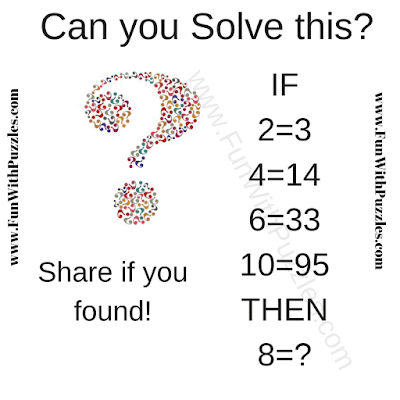Here is the Logical Puzzle Question to test your intelligence. In this puzzle question, there is some equation given in the picture puzzle. These equations are incorrect Mathematically but these are correct logically. Can you decipher the logic of these equations and then solve the last equation?Can you pass this intelligence test?

The answer to this "Intelligence Test Question", can be viewed by clicking on the button. Please do give your best try before looking at the answer.

Unknown said...

54

Rajesh Kumar said...

It will be great if you can write down the logical reasoning used to solve this puzzle along with your answer.

Anonymous said...

60

Logic

2=3(2^2-2/1)
4=14(4^2-4/2)
6=33(6^2-6/2)
10=95(10^2-10/2)
So 8=60(8^2-8/2)

Rajesh Kumar said...

Yes, your answer and logical reasoning to this test question are right.